Related Articles
Text Generation using knowledge distillation and GAN
• Last Updated : 02 Sep, 2020

The most common method for text generation is Recurrent neural network. However, the RNN based text generators use maximum likelihood estimation uses previous observations to predict the next word/sentence. However, Maximum likelihood (MLE) based estimators are simplistic and suffer from exposure bias. In the paper titled “TextKD-GAN: Text Generation using Knowledge Distillation and Generative Adversarial Networks “, presented by researchers at Huawei’s Noah’s Ark Lab. The author explores the uses of GAN in this NLP task and proposed a GAN architecture that does the same.

Knowledge Distillation: Knowledge distillation is a model compression method in which a small model is trained to mimic a pre-trained, larger model (or ensemble of models). This training set is sometimes referred to as “teacher-student”, where the large model is the teacher and the small model is the student. The role of the student model is to mimic the characteristics of larger (teacher) models such as hidden representation, output probabilities, or the sentence (for example) generated by them.

Architecture:

In this paper, the author uses a generative model (GAN) as a student that tries to mimic the output representation of Autoencoder instead of mapping to a one-hot representation of text.

Several methods will be introduced to generate text using GAN, one of them is W-GAN. The problem with W-GAN is that discriminator receives the output from a softmax and one-hot representation, the difference in encoding helps discriminator easily tell the difference between real and generated encoding. This paper deals with the issue by providing a continuous smooth representation of words instead of one-hot and train the discriminator to differentiate between the continuous representations. In this paper, the authors use a conventional autoencoder to replace the one-hot representation with softmax reconstructed output, which is a smooth representation that yields a smaller variance in gradients.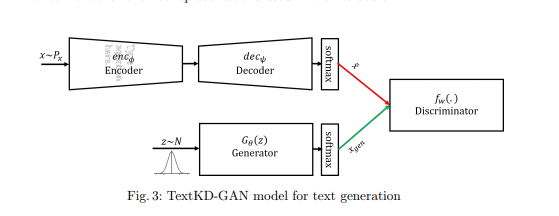For the autoencoder architecture, this paper trains use 512 Long Short Term Memory (LSTM) cells for both the encoder and decoder part. This paper trains first the autoencoder part and then it trains GAN architecture (first discriminator part and then generator part).

Loss function:

The training of Autoencoder and GAN architecture does simultaneously. For this, there is 3 loss function that is used, these are:

• A reconstruction loss term for Autoencoder.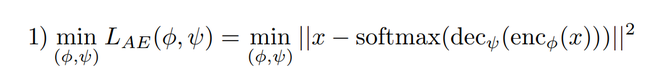• A discriminator loss function with a gradient penalty.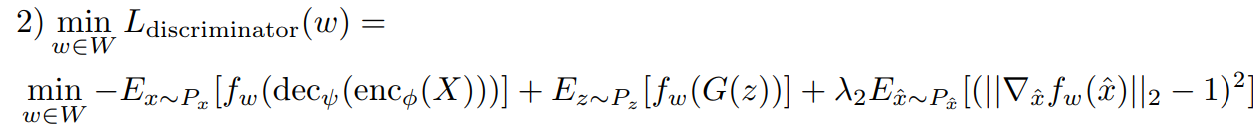• An adversarial GAN loss for generator.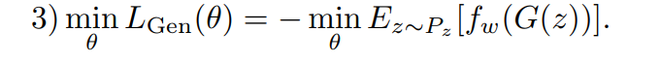Results:

The authors experimented with the model on two different datasets: Stanford Natural Language Corpus (SNLI) and Google 1 billion benchmark language modeling data. The authors use BLEU-N score to calculate and compare the results of the model with previous state-of-the-art architecture. The BLEU score can be calculated as: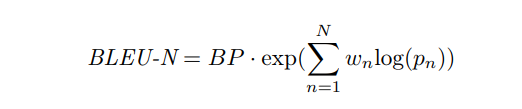where BP is the brevity penalty, pn is the probability of anagram and w = 1/n.

• The BLEU score of different models on Google 1 billion benchmark datasets are as follows: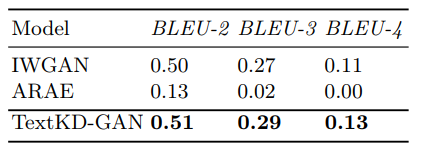• The BLEU-N scores of different models on Stanford Natural Language Inference dataset are as follows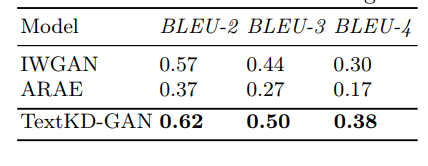Example of text generated using Stanford Natural Language Inference (SNLI) dataset are as follows: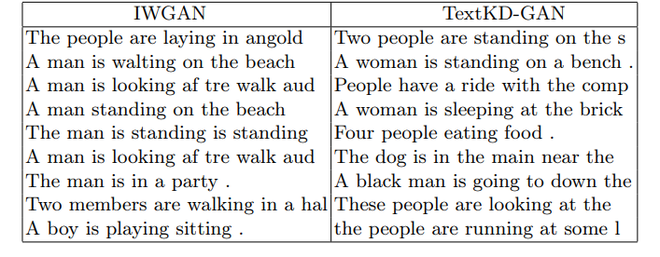Here IWGAN (improved Wasserstein GAN) and ARAE (Adversarially Regularized Autoencoder).

References:My Personal Notes arrow_drop_up
Recommended Articles
Page :